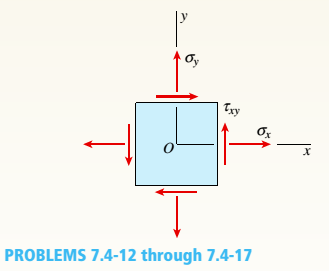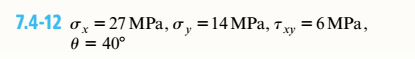# An clement in plane stress is subjected to stresses a,. cry, and (see figure). Using Mohr’s circle, determine the stresses acting on an element oriented at an angle 0 from the x axis. Show these stresses on a sketch of an clement oriented at the angle 0. Now. The angle 0 is positive when count ere clockwise and negative when clockwise.### Mechanics of Materials (MindTap Co...

9th Edition
Barry J. Goodno + 1 other
Publisher: Cengage Learning
ISBN: 9781337093347
Chapter 7, Problem 7.4.12P
Textbook Problem
1 views

## An clement in plane stress is subjected to stresses a,. cry, and (see figure). Using Mohr’s circle, determine the stresses acting on an element oriented at an angle 0 from the x axis. Show these stresses on a sketch of an clement oriented at the angle 0. Now. The angle 0 is positive when count ere clockwise and negative when clockwise.To determine

The values of stress on the element oriented at angle 40°from x-axis using Mohr’s circle.

### Explanation of Solution

Given information:

The normal stress along x-axis is 27MPa, normal stress along y-axis is 14MPa,and shear stress along xy-plane is 6MPa, orientation of element is 40°.

The following are the steps TO-Draw the Mohr’s circle

Draw the x-axis as normal stress and y-axis as shear stress and indicate the origin as point O (0,0).

1. Write the expression to locate the center point C of the circle from the origin O(ie., OC).
2. OC=σavg=σx+σy2        …… (I)

Here, the normal stress along x-axis is σx, normal stress along y-axis is σy, and average stress is σavg.

3. Mark the center point as C: (σavg,0).
4. Write the expression for the radius of Mohr’s circle
5. R=(σxσavg)2+τxy2        …… (II)

Here, the radius of the Mohr’s circle is Rand shear stress along xy-plane is τxy.

6. Write the expression for principle angle.

θp=12tan1(τxyσxσavg)        …… (III)

Here, principle angle is θp.

Write the expression for the stress acting on element along x-direction.

σx=σavg+Rcos(2θ2θp)        …… (IV)

Here, the stress acting on element along x-direction is σxand orientation of the plane is θ.

Write the expression for the stress acting on element along y-direction.

σy=σavgRcos(2θ2θp)        …… (V)

Here, the stress acting on element along y-direction is σy.

The shear stress acting on element along x-y plane.

τxy=Rsin(2θ2θp)......VI)

Here, shear stress acting on element along x-y plane is τxy.

Calculation:

Substitute 27MPafor σxand 14MPafor σyin Equation (I).

σavg=27MPa+14MPa2=27MPa2=20.50MPa

Substitute 27MPafor σavgand 20.50MPafor σyand 6MPafor τxyin Equation (II).

R=(27MPa20.5MPa)2+(6MPa)2=78

### Still sussing out bartleby?

Check out a sample textbook solution.

See a sample solution

#### The Solution to Your Study Problems

Bartleby provides explanations to thousands of textbook problems written by our experts, many with advanced degrees!

Get Started

Find more solutions based on key concepts
What is middleware?

Principles of Information Systems (MindTap Course List)

How do you translate business rules into data model components?

Database Systems: Design, Implementation, & Management

In the U.S., the fuel consumption of an automobile is expressed in X miles per gallon. Obtain a single factor t...

Engineering Fundamentals: An Introduction to Engineering (MindTap Course List)

Determine the largest angle for which the homogeneous block remains at rest.

International Edition---engineering Mechanics: Statics, 4th Edition

What e input masksâ€� What are validation rules? Why are they important?

Systems Analysis and Design (Shelly Cashman Series) (MindTap Course List)

What steps can you take to detect keyboard monitoring software?

Enhanced Discovering Computers 2017 (Shelly Cashman Series) (MindTap Course List)

Voltage can be described as ____________ .

Welding: Principles and Applications (MindTap Course List)

If your motherboard supports ECC DDR3 memory, can you substitute non-ECC DDR3 memory?

A+ Guide to Hardware (Standalone Book) (MindTap Course List)